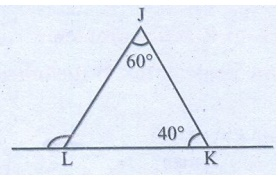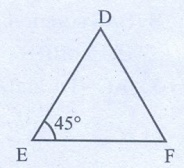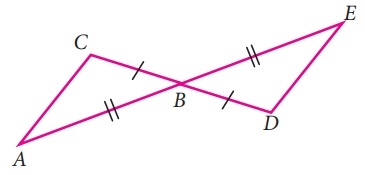Home | | Maths 7th Std | Exercise 4.3

# Exercise 4.3

7th Maths : Term 2 Unit 4 : Geometry : Exercise 4.3 : Miscellaneous Practice problems, Challenge Problems, Text Book Back Exercises Questions with Answers, Solution

Exercise 4.3

Miscellaneous Practice problems

1. In an isosceles triangle one angle is 76°. If the other two angles are equal find them.

Solution:

In an isosceles triangle one angle = 76°

Sum of three angles = 180°

The other two equal angles = 180° – 76°

= 104°

One angle = 104/2 = 52°

The other two equal angles are 52°, 52°.

2. If two angles of a triangle are 46° each, how can you classify the triangle?

Solution:

If two angles are equal Corresponding two sides also equal. So it is an isosceles triangle.

3. If one angle of a triangle is equal to the sum of the other two angles, find the type of the triangle.

Solution:

One angle = Sum of two angles

90° = 45°+ 45°

So, the triangle is the right angled triangle.

4. If the exterior angle of a triangle is 140° and its interior opposite angles are equal, find all the interior angles of the triangle.

Solution:

The exterior angle of a triangle = 140°

The exterior angle = Sum of two interior opposite angles. The interior opposite angles are equal.

The sum of two interior oppositeangles = 140°

One angle = 140 / 2 = 70o

Third angle = 180° – 140° = 40°

The three angles = 70°, 70°, 40°

5. In ∆JKL, if J = 60° and K = 40°, then find the value of exterior angle formed by extending the side KL.

Solution:

The exterior angle = Sum of two interior opposite angles.

The exterior angle L = 60° + 40° = 100°6. Find the value of ‘x’ in the given figure.Solution:

The exterior angle ABD = 128°

The exterior angle = Sum of two interior opposite angles.

128° = BCD + BDC

100° + BDC = 128°

BDC = 128° – 100° = 28°

The inner angle BDC = 28°

BDC + x = 180° (Linear angles)

28° + x =180°

x = 180° – 28° = 152°

x = 152°

7. If ∆MNO DEF, M = 60° and E = 45° then find the value of O.

Solution:

∆MN0 ∆DEF

M = D, N = E, O = F

E = 45°

N = 45°Sum of three angles of a triangle = 180°

M + N + O = 180°60° + 45°+ O = 180°

105° + O = 180°

O = 180° – 105° = 75°

O = 75°

8. In the given figure ray AZ bisects BAD and DCB, prove that (i) ∆BAC DAC (ii) AB = AD.Solution:

From the given figure,

In ∆ BAC and ∆ DAC,

The ray AZ is the angle bisector of A and C

BAC = DAC

BCA = DCA

AC is common By

ASA criterion

∆ BAC ∆ DAC

∆BAC and ∆ DAC are congruent triangles, then their corresponding parts are congruent. AB = AD.

9. In the given figure FG = FI and H is midpoint of GI, prove that ∆FGH FHISolution:

From the figure, In ∆FGH and ∆FHI,

H is the mid point of GI.So, GH = HI

FG = FI (Given)

FH is common

By SSS criterion, ∆FGH ∆FHI

10. Using the given figure, prove that the triangles are congruent. Can you conclude that AC is parallel to DE.Solution:

From the figure, In ∆ ABC and ∆ BDE,

AB = BE

BC = BD

ABC = DBE (Vertically opposite angles)

By SAS criterion,

∆ABC   ∆BDE

So, AC = DE and AC | | DE

Challenge Problems

11. In given figure BD = BC, find the  value of x.Solution:

In ∆ ABC, Exterior angle C = 115°

BCD + 115° = 180° (Linear angles)

BCD = 180°–115° = 65°

BD = BC (Given)

So, BCD = BDC

BCD = 65°

So, BDC = 65°

The exterior angle of BAD is ∆BDC.

The exterior angle BDC = The sum of two interior opposite angles.

65° = 35° + x

x = 65°– 35° = 30°

12. In the given figure find the value of x.Solution:

From the figure,

The exterior angle of ∆LMN is KML.

So the exterior angle KML = Sum of two interior opposite angles.

= MLN + MNL

= 26° + 30° = 56°

The exterior angle of ∆KMJ is KJL.

So, the exterior angle KJL = Sum of two interior opposite angles,

x = JKM + KMJ

= 58° + 56° = 114°

x = 114°

13. In the given figure find the values of  x and y.Solution:

From the figure,

The exterior angle BAX = Sum of two interior opposite angles.

62°. = ABC + ACB

62° = 28° + x

x = 62° – 28 = 34o

x = 34°

CAB + BAX = 180° (Linear angles)

y + 62° = 180°

y = 180°– 62° = 118°

y = 118°

x = 34°

y = 118°

14. In ∆DEF, F = 48°, E = 68° and bisector of D meets FE at G. Find FGD.Solution:

From the figure,

In ∆DEF,

D + E + F = 180° (Sum of three angles of a triangle)

D + 68° + 48° = 180°

D +116° = 180°

D = 180° – 116° = 64°

Angle bisector of D is DG,

So, FDG = EDG ………… (1)

FDG + EDG = 64°

From equation ………. (1)

EDG + EDG = 64°

2 EDG = 64°

EDG = 32°

The exterior angle FGD = Sum of two interior opposite angles.

= DEG + EDG

FGD = 68° + 32° = 100°

FGD = 100°

15. In the figure find the value of x.Solution:

From the figure,

The exterior angle UTP = Sum of two interior opposite angles.

105° = TSP + TPS

105° = 75° + TPS

TPS = 105° – 75° = 30°

TPS + TPR + RPQ = 180° (Linear angles)

30° + 90° + RPQ = 180°

120° + RPQ = 180°

RPQ =180° – 120° = 60°

RPQ = 60o

VRQ + QRP = 180o (Linear angles)

145o + QRP = 180°

QRP = 180 – 145° = 35°

The exterior angle PQY = Sum of two interior opposite angles.

QRP + RPQ

x = 60o + 35o = 95°

x = 95°

16. From the given figure find the value of ySolution:

From the figure,

YCX =48°

YCX = ACB (Vertically opposite angles)

ACB = 48°

The exterior angle CBD = Sum of two interior opposite angles.

= BAC + ACB = 57° + 48° = 105°

CBD = CBE + EBD

105° = 65° + EBD

EBD = 105° – 65° = 40°

The exterior angle BDZ = The two interior opposite angles.

BED + EBD

y = 97° + 40° = 137°

y = 137°

Exercise 4.3

1. 52°, 52°

2. Isoceles triangle

3. Right angled triangle

4. 40°, 70°, 70°

5. 100°

6. 152°

7. O = 75º

10. (SAS), ∆CAB ∆EBD ; AC || DE

Challenge problems

11. x = 30º

12. x = 114º

13. x = 34º; y = 118º

14. 100°

15. 95°

16. y = 137º

Tags : Questions with Answers, Solution | Geometry | Term 2 Chapter 4 | 7th Maths , 7th Maths : Term 2 Unit 4 : Geometry
Study Material, Lecturing Notes, Assignment, Reference, Wiki description explanation, brief detail
7th Maths : Term 2 Unit 4 : Geometry : Exercise 4.3 | Questions with Answers, Solution | Geometry | Term 2 Chapter 4 | 7th Maths